# Logistic Growth Models

Vratika Chaudhary
Feb 2019

### Recap

Last two labs we projected populations, but assumed

• Exponential growth model: 'r' is constant

• Stochastic growth model: 'r' is a random variable

• This week we say that 'r' is regulated by the population density

### Resources are limited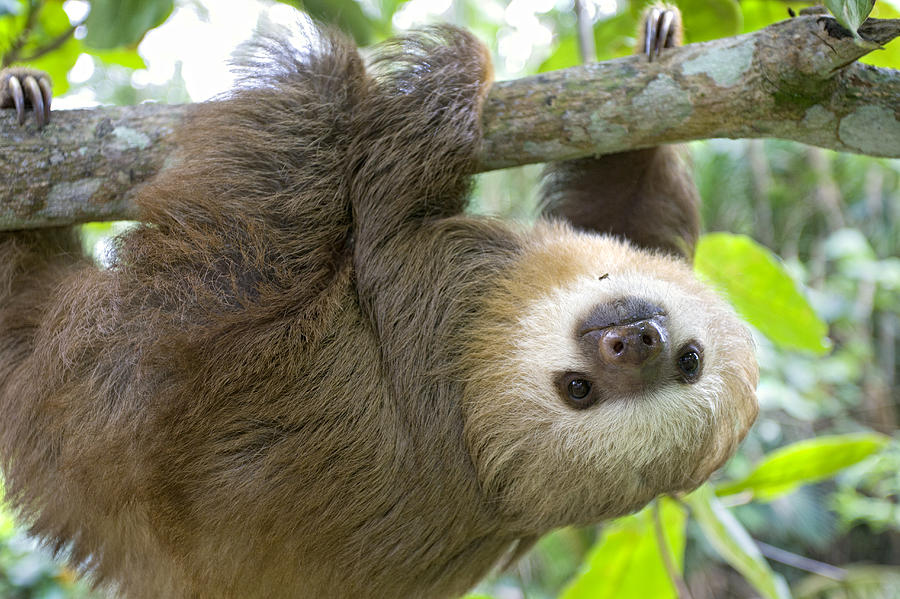### There are only so many babies one can feed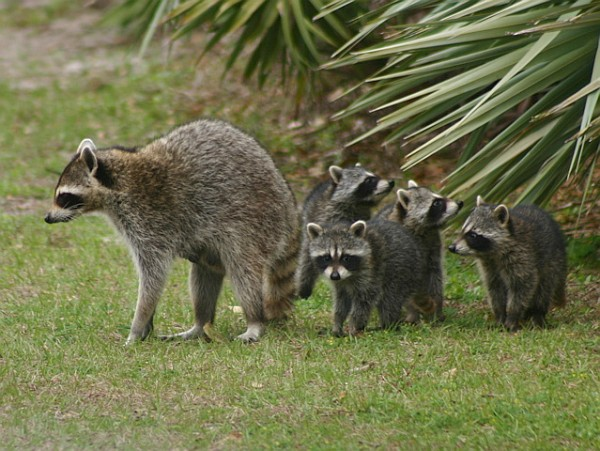### Density-dependent/Logistic growth (Page 49)

Paramters: Logistic growth models assume linear density-dependence of 'r' Every population has a 'K', and when N = K, 'r' ~ 0

-K = carrying capacity

-N= Population size

-'r' = per capita growth rate

-r max= maximum growth rate

### Density-dependent growth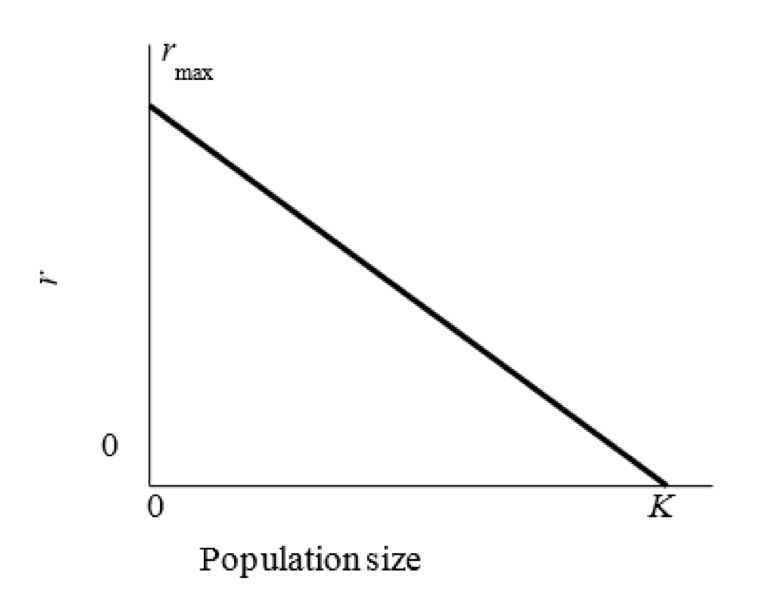### Density-dependent growth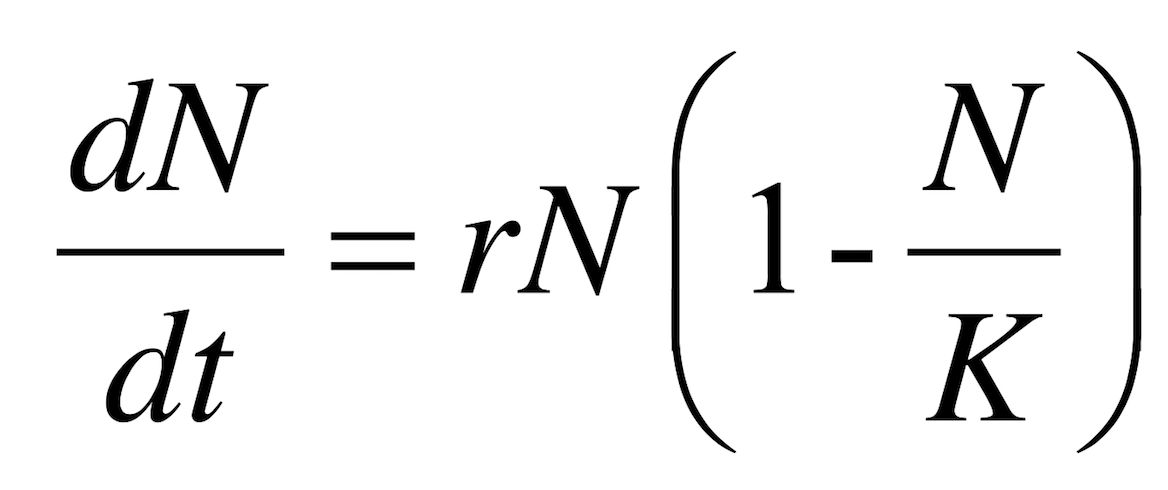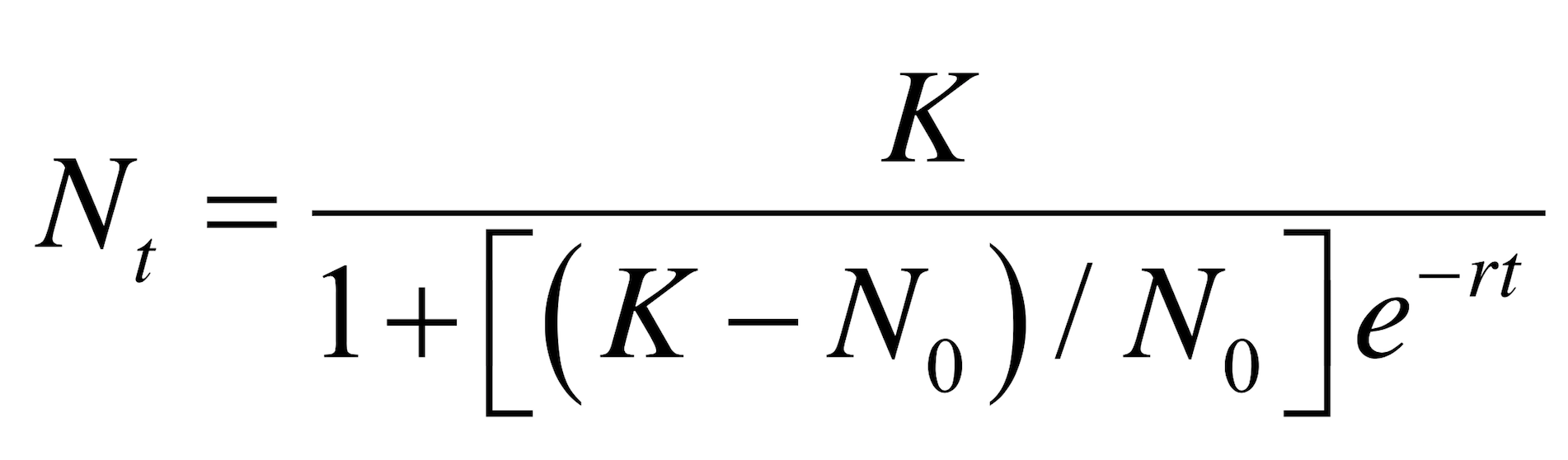### Example: Australian fox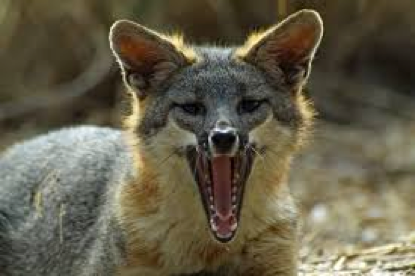### Example Fox data

``````###Create some fake population data, of let's say newly introduced fox population
t = (seq(1900,1920,1)) #####time vector
N = (sort(round((runif(21,200,2489)),0), decreasing = F) )###population vector, rounded and sorted in ascending order
pop<- as.data.frame(cbind(t,N)) ###bind columns
``````
``````     t   N
1 1900 201
2 1901 214
3 1902 243
4 1903 319
5 1904 664
6 1905 689
``````

### See if the population is growing as 'density-dependent'

Page 50

``````##############use ratio method to find 'r'
len=length(pop\$t)
##calculate r as r = log(Nt+1/Nt)
r1= pop\$N[-1]/pop\$N[-len]
r=log(r1)
r
``````
``````  0.062671107 0.127085428 0.272129659 0.733091047 0.036959122
 0.255980192 0.029886010 0.075586859 0.074974273 0.193051774
 0.132370626 0.001354096 0.158586988 0.035728212 0.024216924
 0.007044191 0.127574159 0.010401985 0.014012373 0.011987234
``````

### Fit linear regression

Page 50

``````y=r##where y=r, x= pop\$N[1len-1]
x= pop\$N[1:len-1]
fit.lm= lm(y~x)
plot(x,y,xlab='Population', ylab='Population growth rate')
abline(fit.lm)
#####looking at the plot it looks like this population has a logistic growth trend
``````

### Linear regression plot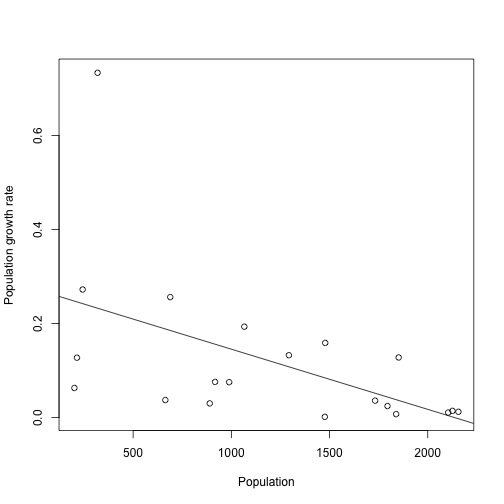### Fit rmax and k

Page 52

``````#######find rmax and K
rmax= fit.lm\$coefficients
rmax
``````
``````(Intercept)
0.2732159
``````
``````slope= fit.lm\$coefficients
##############
#K #carrying capacity
K = -rmax/slope
K
``````
``````(Intercept)
2133.029
``````

### Now we will see how well the model fits our data

We will project our population using logistic growth model (page 53)

``````N0= pop\$N #starting population
time= 1:20
N_t = rep(NA, length(t))##create a empty vector to store results
N_t=N0
###########
``````

### Write your own function to project population

You can give different values of N0, R, K and time (page 46)

``````c.logist<- function(N0,r,K,time){
N_t = rep(NA, length(time))#empty vector
N_t = N0 #initial pop size
for (i in 2: length (time)){
N_t[i] = K / (1 + ((K - N0) / N0) * exp(-r * time[i]))
}

return(N_t)
}
``````

### Use function on the data (Page 53)

``````N_t= c.logist(N0=N0, r=rmax,time=time,K=K)
``````

### How well does the model perform?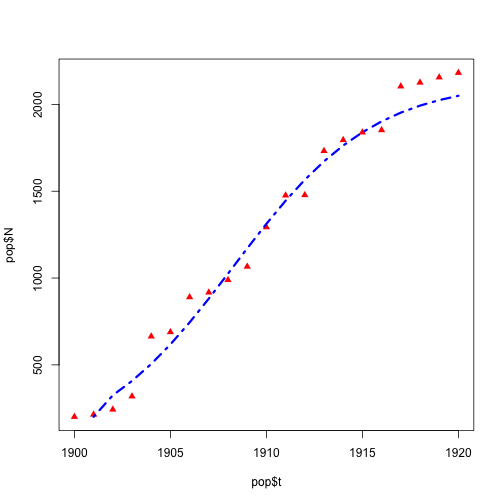### Quiz

Opens at 10:30, will close on Thursday 14th Feb at 5:00 PM